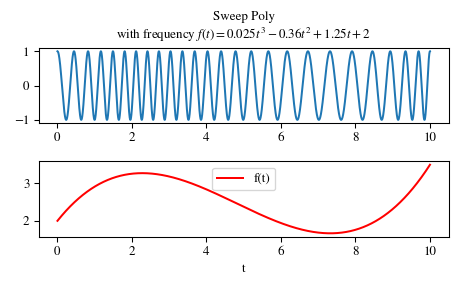# scipy.signal.sweep_poly¶

scipy.signal.sweep_poly(t, poly, phi=0)[source]

Frequency-swept cosine generator, with a time-dependent frequency.

This function generates a sinusoidal function whose instantaneous frequency varies with time. The frequency at time t is given by the polynomial poly.

Parameters: t : ndarray Times at which to evaluate the waveform. poly : 1-D array_like or instance of numpy.poly1d The desired frequency expressed as a polynomial. If poly is a list or ndarray of length n, then the elements of poly are the coefficients of the polynomial, and the instantaneous frequency is f(t) = poly*t**(n-1) + poly*t**(n-2) + ... + poly[n-1] If poly is an instance of numpy.poly1d, then the instantaneous frequency is f(t) = poly(t) phi : float, optional Phase offset, in degrees, Default: 0. sweep_poly : ndarray A numpy array containing the signal evaluated at t with the requested time-varying frequency. More precisely, the function returns cos(phase + (pi/180)*phi), where phase is the integral (from 0 to t) of 2 * pi * f(t); f(t) is defined above.

Notes

New in version 0.8.0.

If poly is a list or ndarray of length n, then the elements of poly are the coefficients of the polynomial, and the instantaneous frequency is:

f(t) = poly*t**(n-1) + poly*t**(n-2) + ... + poly[n-1]

If poly is an instance of numpy.poly1d, then the instantaneous frequency is:

f(t) = poly(t)

Finally, the output s is:

cos(phase + (pi/180)*phi)

where phase is the integral from 0 to t of 2 * pi * f(t), f(t) as defined above.

Examples

Compute the waveform with instantaneous frequency:

f(t) = 0.025*t**3 - 0.36*t**2 + 1.25*t + 2


over the interval 0 <= t <= 10.

>>> from scipy.signal import sweep_poly
>>> p = np.poly1d([0.025, -0.36, 1.25, 2.0])
>>> t = np.linspace(0, 10, 5001)
>>> w = sweep_poly(t, p)


Plot it:

>>> import matplotlib.pyplot as plt
>>> plt.subplot(2, 1, 1)
>>> plt.plot(t, w)
>>> plt.title("Sweep Poly\nwith frequency " +
...           "$f(t) = 0.025t^3 - 0.36t^2 + 1.25t + 2$")
>>> plt.subplot(2, 1, 2)
>>> plt.plot(t, p(t), 'r', label='f(t)')
>>> plt.legend()
>>> plt.xlabel('t')
>>> plt.tight_layout()
>>> plt.show()#### Previous topic

scipy.signal.square

#### Next topic

scipy.signal.unit_impulse